## Friction definition

If we push the book on the table at any speed, then the book comes to rest after running a little. This makes it clear that a force acts on the book which opposes its motion across the table. This force is called the friction force.
In fact, whenever the surface of one body slips at the bottom of another body, each body exerts frictional force on the other body. That is parallel to the contact plane of the body.
This force is applied to each body in the direction of its relative motion. In this way, when a book goes from left to right and on the floor of the table, the force of friction on the book is towards left. The force of friction between two planes can exist even before the relative motion starts.

## Friction Example

Suppose a block on the hefty floor of a table is in resting state.There are two forces on the block.Its weight is mg which is the vertical downward gaze at its center of gravity. And the reaction force P charged by this table is the vertical upward direction. P=mg
When we apply a small horizontal force F to the right (considered) on the block (Fig. 1b), the block does not start moving from its position.  Now, the force P exerted on the block by the floor of the table bends to the left msin so that P, mg and F can form a closed triangle (since the block is still in equilibrium).  Force P can be divided into two components: parallel to the plane and perpendicular to the plane. The parallel component of the connections is called ‘static friction
And this balances the applied force R.  The reaction on the block, the vertical component of the contact table, is R, which balances the weight mg of the block.

#### You may also like

••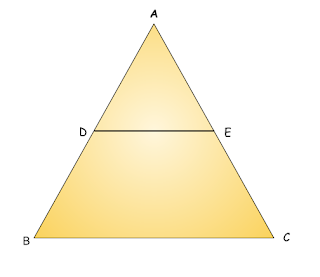•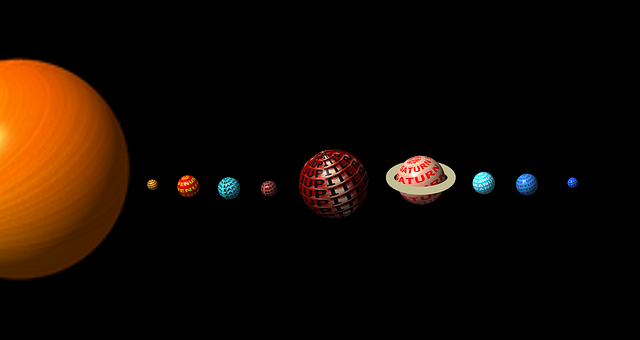•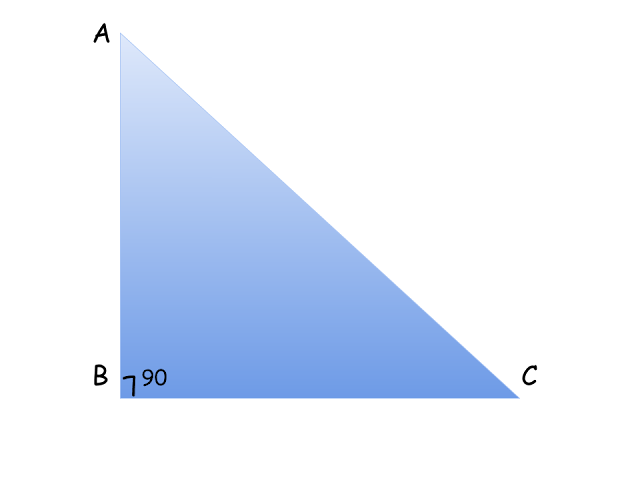•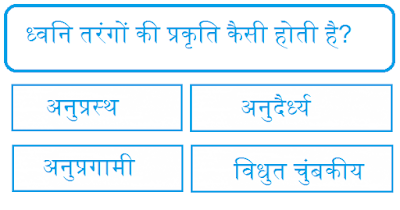•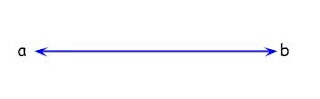••••